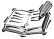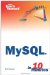# Aggregate Functions

MySQL provides five aggregate functions that enable you to summarize table data without retrieving every row. By using these functions, you can find the total number of rows in a dataset, the sum of the values, or the highest, lowest, and average values.

#### The COUNT() Function

The COUNT() function counts values in a query. You can perform a count on a single column by putting the column name in parentheses. More commonly, though, you use COUNT(*) to return the total number of rows returned by a query.

The following example counts the total number of orders that have been placed in the sample database:

` mysql> SELECT COUNT(*)     -> FROM orders; +----------+ | COUNT(*) | +----------+ |        7 | +----------+ 1 row in set (0.00 sec) `## NULL Values

When COUNT() is called with a column name, it counts only the number of rows that have a value in that column. If the data in that column is NULL, it is not counted.

To find the number of unique values in a column, use COUNT() with the DISTINCT keyword. The following example finds the total number of customer contacts in the database and also the number of unique last names:

` mysql> SELECT COUNT(*), COUNT(DISTINCT last_name)     -> FROM customer_contacts; +----------+---------------------------+ | COUNT(*) | COUNT(DISTINCT last_name) | +----------+---------------------------+ |       11 |                        10 | +----------+---------------------------+ 1 row in set (0.02 sec) `

#### The SUM() Function

The SUM() function computes a total from the data in a specified column. For example, you can use the following query to return the total number of units ordered of a particular product:

` mysql> SELECT SUM(quantity)     -> FROM order_lines     -> WHERE product_code = 'MAXI'; +---------------+ | SUM(quantity) | +---------------+ |            48 | +---------------+ 1 row in set (0.00 sec) `

If you include an expression within SUM(), it is evaluated for each row of data and the total result is returned, as shown in the following query:

` mysql> SELECT SUM(quantity + 1)     -> FROM order_lines     -> WHERE product_code = 'MAXI';  +-------------------+ | SUM(quantity + 1) | +-------------------+ |                54 | +-------------------+ 1 row in set (0.00 sec) `

You can use SUM() with a conditional expression to return the number of rows that meet the condition. Because the expression evaluates to 1 each time the condition is true and 0 each time it is false, the following query finds the number of products that weigh less than 5 pounds:

` mysql> SELECT SUM(weight < 5)     -> FROM products; +-----------------+ | SUM(weight < 5) | +-----------------+ |               2 | +-----------------+ 1 row in set (0.00 sec) `

#### The AVG() Function

You can use the AVG() function to compute an average of the data in a specified column. The following query returns the average weight of all the items in the products table:

` mysql> SELECT AVG(weight)     -> FROM products; +-------------+ | AVG(weight) | +-------------+ |    4.666667 | +-------------+ 1 row in set (0.00 sec) `

The AVG() function computes a mean average. The same result could be performed using SUM() and COUNT(), as follows:

` mysql> SELECT SUM(weight) / COUNT(weight)     -> FROM products; +-----------------------------+ | SUM(weight) / COUNT(weight) | +-----------------------------+ |                      4.6667 | +-----------------------------+ 1 row in set (0.00 sec) `## NULL Values

NULL values are excluded when computing an average with AVG().

#### The MIN() and MAX() Functions

The MIN() and MAX() functions return the smallest and greatest values from a column, respectively. The following query finds the least expensive product in the database:

` mysql> SELECT MIN(price)     -> FROM products; +------------+ | MIN(price) | +------------+ |       5.99 | +------------+ 1 row in set (0.00 sec) `

The next query finds the date of the most recent order placed by using MAX() on the date_ordered column of the orders table.

` mysql> SELECT MAX(order_date)     -> FROM orders; +-----------------+ | MAX(order_date) | +-----------------+ | 2006-02-05      | +-----------------+ 1 row in set (0.01 sec)  `Sams Teach Yourself MySQL in 10 Minutes
ISBN: 0672328631
EAN: 2147483647
Year: 2006
Pages: 165
Authors: Chris Newman

Similar book on Amazon

flylib.com © 2008-2017.
If you may any questions please contact us: flylib@qtcs.net